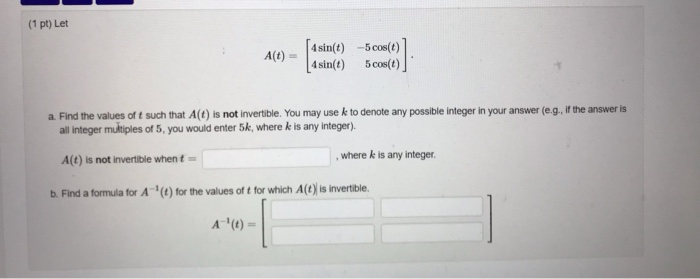# Solved: (1 Pt) Let 4sin(t) -5cos(t) 4sin(t) A(t) 5 Cos(t) A. Find The Values Of T Such That A(t) Is Not Invertible. You May Usek To Denote Any Possible Integer In Your Answer (e.g, If The Answer Is Al

By |(1 pt) Let 4sin(t) -5cos(t) 4sin(t) A(t) 5 cos(t) a. Find the values of t such that A(t) is not invertible. You may usek to denote any possible integer in your answer (e.g, if the answer is all integer multiples of 5, you would enter 5k, where k is any integer). ,where k is any integer A(t) is not invertible when t b. Find a formula for A(t) for the values of t for which A(t) is invertible A (0)